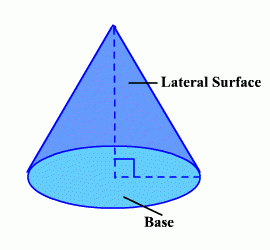# Find the surface area of a cone with a slant height of 11 and a radius of 15?

The total surface area of a cone is the sum of the area of its base and the lateral (side) surface.So:

Area of a cone = (area of the base) + (area of the side) = (pi)r^2 + (pi)(r)(l)

Recall that the area of a circle (the base) is πr2 and you add that to the lateral area (side of the cone) which is πrl in this case.

A = π(15)^2 + π(15)(11) = 225π + 165π = 390π units^2.

thanked the writer.